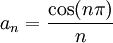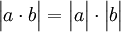# Example Relating Sequences of Absolute Values

Previous: Relationship to Sequences of Absolute Values

Next: Squeeze Theorem for Sequences

## Example

Determine whether the sequenceconverges.

## Complete Solution

We can quickly determine that the sequence converges by noting thatTherefore, by our theorem for the relationship to sequences of absolute values, the given sequence converges.

## Explanation of Each Step

### Step (1)

In Step (1), we asserted our theorem for the relationship between sequences of absolute values.

### Step (2)

In this step, we used a property of absolute values:for any real numbers a and b.

### Step (3)

Our trigonometric function has the property that, and so## Possible Challenges

### Getting Started

It is not always obvious to know which theorem to apply to test for convergence. In this case, we had a specific trigonometric function,Recognizing that this function takes on values of ±1 gives us a hint that we could try using the relationship to sequences of absolute values, because the absolute value would eliminate the cosine function.

Alternatively, we could have also used the Squeeze Theorem to solve this example, which we explore next.

Previous: Relationship to Sequences of Absolute Values

Next: Squeeze Theorem for Sequences